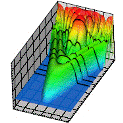## Research Papers in Physics and Astronomy## Anthony F. Starace Publications

1-1-2002

#### Comments

Published in Journal of Physics B: Atomic, Molecular and Optical Physics 35 (2002), pp. 77–91. Online at http://stacks.iop.org/JPhysB/35/77 Copyright © 2002 Institute of Physics Publishing Ltd. Used by permission.

#### Abstract

A formal multipole expansion of tensor functions dependent on several vector parameters is obtained in an invariant differential form. We apply this result to derive multipole expansions of finite rotation matrices in terms of finite sums of bipolar harmonics. The multipole expansion in terms of bipolar harmonics of unit vectors r1 and r2 are analyzed for functions of the type f (r)Ylm( r) (with r = r1 − r2), which are important in two-center problems. As another example of the application of the multipole expansion technique, reduction formulae are obtained for tensor constructions which appear in analyses of angular distributions in photoprocesses which take exact account of non-dipole (or retardation) effects. As a result, the “photon factors” in the angular distribution for an arbitrary photoprocess are expressed in an invariant form involving only the photon polarization vector and spherical harmonics of the photon wavevector.

COinS